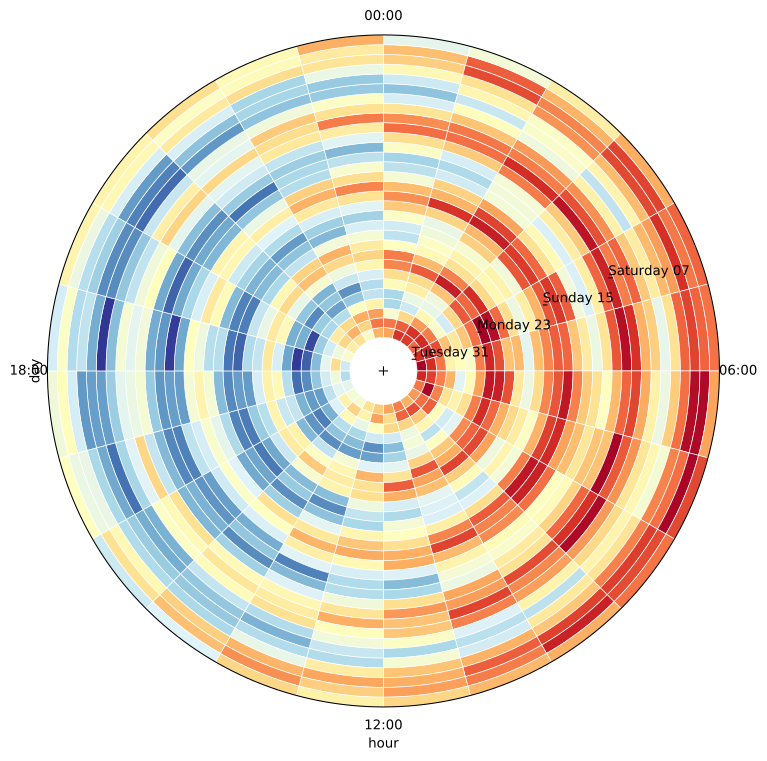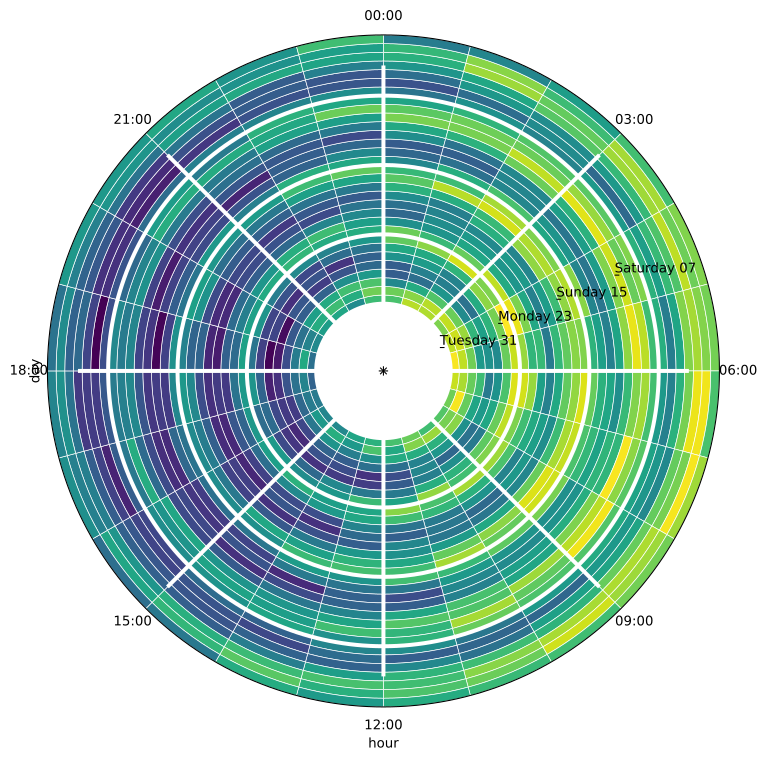# Radialheatmap¶

Title
HeatMap Element (radial)
Dependencies
Matplotlib
Backends
Matplotlib
Bokeh
In :
import numpy as np
import pandas as pd
import holoviews as hv
from holoviews import opts

hv.extension('matplotlib')

hv.output(fig='svg')
opts.defaults(opts.HeatMap(fig_size=250))A radial HeatMap is well suited to discover periodic patterns and trends in time series data and other cyclic variables. A radial HeatMap can be plotted simply by activating the radial plot option on the HeatMap element.

Here we will create a synthetic dataset of a value varying by the hour of the day and day of the week:

In :
days = 31
hours = 24
size = days*hours

def generate_hourly_periodic_data(x):
periodic_weekly = np.sin(x*2*np.pi / (24*7))
periodic_daily = np.sin(x*2*np.pi / 24)
noise = np.random.random(size=x.size)
return periodic_weekly + periodic_daily + noise

x = np.linspace(0, size, size)
y = generate_hourly_periodic_data(x)

date_index = pd.date_range(start="2017-10-01", freq="h", periods=size)
kdim_segment = date_index.strftime("%H:%M")
kdim_annular = date_index.strftime("%A %d")

df = pd.DataFrame({"values": y, "hour": kdim_segment, "day": kdim_annular}, index=date_index)


As with a regular HeatMap the data should consist of two index variables or key dimensions and one or more value dimensions. Here we declare the 'hour' and 'day' as the key dimensions. For a radial HeatMap to make sense the first key dimension, which will correspond to the radial axis, should be periodic. Here the variable is 'hour', starting at midnight at the top:

In :
hv.HeatMap(df, ["hour", "day"]).opts(radial=True)

Out:The resulting plot is quite bare so we may want to customize it, there are a number of things we can do to make the plot clearer:

1. Increase the inner padding with the radius_inner option.
2. Increase the number of ticks along the radial axis using xticks
3. Add radial separator marks with the xmarks option.
4. Change the colormap using the cmap style option.
In :
hv.HeatMap(df, ["hour", "day"]).opts(
opts.HeatMap(cmap='viridis', radial=True, radius_inner=0.2, xmarks=8, xticks=8, ymarks=4))

Out:Download this notebook from GitHub (right-click to download).